Next: Free-Particle Solutions and Wave Up: Dirac Equation Previous: Time Reversal

# Combined PCT Symmetry

Recall the parity transformation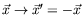,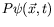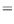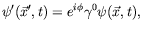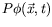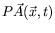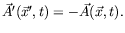(5.262)

The parity transformation leaves the Dirac equation and all physical observables unchanged.

We now combine all three symmetries,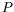,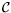and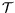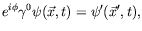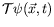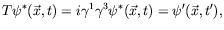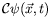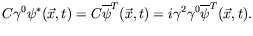(5.263)

to obtain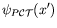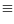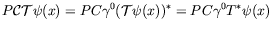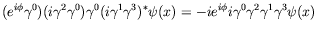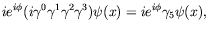(5.264)

with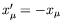.

Since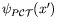can represent a positron, we see that it is an electron moving backward in space-time and multiplied by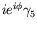. Thus positrons are negative-energy electrons running backward in space-time. This is the basis of the Stückelberg-Feynman form of positron theory.

For a free-particle spin-momentum eigenstate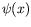and negative energy, we see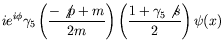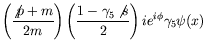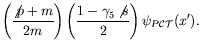(5.265)

Therefore a positron wave function is a negative-energy electron moving backward in time, multiplied by.

For an arbitrary solution in the presence of electromagnetic forces, the negative energy eigenvalue equation is(5.266)

Carrying out the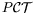transformation gives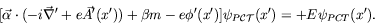(5.267)

Notice that since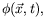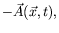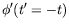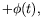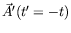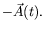(5.268)

Therefore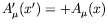.Next: Free-Particle Solutions and Wave Up: Dirac Equation Previous: Time Reversal
Douglas M. Gingrich (gingrich@ ualberta.ca)
2004-03-18